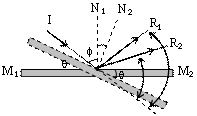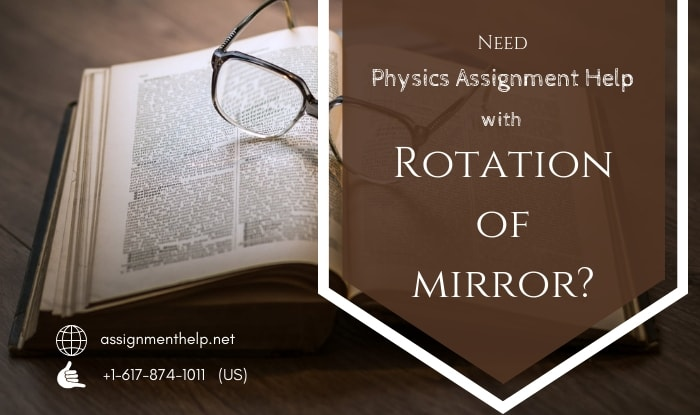# Physics Assignment Help With Rotation of mirror

1. Home
2. Physics
3. Light
4. Rotation Of Mirror

## 13.1.2 Rotation of mirror

Let f be angle of incidence in first position of mirror M1 M2. Further, let mirror is rotated through angle q, then normal will also rotate by same angle is same sense.

d1 = Deviation in first position of mirror

= p – 2f

d2 = Deviation in second position of mirror

= p – 2 (q + f)

\ d1 – d2 = p – 2f – p – 2(q + f) = 2qThus, the angle turned by the reflected ray is twice the angle turned by the mirror.

### Email Based Assignment Help in Rotation of mirror

We are the leading online Assignment Help provider. Find answers to all of your doubts regarding the Rotation of mirror. Assignmenthelp.net provide homework, Assignment Help to the school, college or university level students. Our expert online tutors are available to help you in Rotation of mirror. Our service is focused on: time delivery, superior quality, creativity and originality.

To submit physics Rotation of mirror assignmentupload assignmentFollowing are some of the areas in physics Light which we provide help: